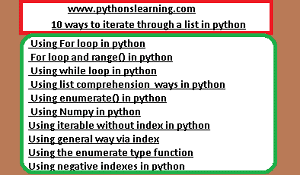# 10 ways to iterate through a list in python

The list is similar to array in other languages except for python, which provides the extra benefit of being dynamic in size nature. In Python, the list is a type of  Data Structures, which is used to store different and multiple kinds of data at the same time.10 ways to iterate through a list in python

## There are many ways to iterate over a list in Python language. Let’s see all different ways in python:

1.  Using For loop in python
2.  For loop and range() in python
3.  Using while loop in python
4.  Using list comprehension  ways in python
5.  Using enumerate() in python
6.  Using Numpy in python
7. Using iterable without index in python
8. Using general way via index
9. Using the enumerate type function
10. Using negative indexes in python

### Method #1: Using For loop in python

# Python3 code to iterate over a list in python
list = [1, 3, 5, 8, 9]
# Using for loop
for n in list:
print(n)

Output:

1
3
5
8
9

#### Method #2: For loop and range() in python

In case we want to use the traditional For loop and range() in python which iterates from number x to number y.

# Python3 code to iterate over a list in python
num = [1, 3, 5, 8, 9]
# getting length of list in python
length = len(num)
# How to Iterating the index
for i in range(length):
print(list[i])

learn pandas in python
Output:

1
3
5
8
9

Method #3: Using while loop in python

# Python3 code to iterate over a list in python
num = [1, 3, 5, 8, 9]
# Getting length of list in python
length = len(num)
i = 0
# Iterating using while loop in python
while i < length:
print(num[i])
i += 1

Output:

1
3
5
8
9

Method #4: Using list comprehension  ways in python

# Python3 code to iterate over a list in python
num = [1, 3, 5, 8, 9]
# Using list comprehension in python
[print(i) for i in num]

Output:

1
3
5
8
9

Method #5: Using enumerate() in python
suppose if we want to convert the list of set into an iterable list of tuples, then you can use the enumerate() function in python.

# Python3 code to iterate over a list in python
num = [1, 3, 5, 8, 9]
# Using enumerate() function in python
for i, val in enumerate(num):
print (i, “,”,val)

Output:

0 , 1
1 , 3
2 , 5
3 , 8
4 , 9

Method #6: Using Numpy in python
If in code we provide n-dimensional lists, then it is sometimes better to use an external library (NumPy)
filter_none

# Python program for using numpy func.
# iterating over array in python
import numpy as geek
z = z.reshape(3, 3)
# iterating an array in python
for y in geek.nditer(z):
print(y)

Output:

0
1
2
3
4
5
6
7
8

Method 7# − Using iterable without index in python
Example
sach_list = [‘p’,’y’,’t’,’h’,’o’,’s’,’l’,’e’,’a’,’r’,’n’,’i’,’n’,’g’]

# Iterate over the list in python
for value in sach_list:
print(value, end=”)
output:
pythonslearning

Method 8# − Using general way via index
Example
sach_list = [‘p’,’y’,’t’,’h’,’o’,’s’,’l’,’e’,’a’,’r’,’n’,’i’,’n’,’g’]

# Iterate over the list in python
for value in range(0,len(sach_list)):
print(sach_list[value], end=”)
output:
pythonslearning

Method 9# − Using the enumerate type function
Example
sach_list = [‘p’,’y’,’t’,’h’,’o’,’s’,’l’,’e’,’a’,’r’,’n’,’i’,’n’,’g’]

# Iterate over the list in python
for value,char in enumerate(sach_list):
print(char, end=”)
output:
pythonslearning

Method 10# − Using negative indexes in python
Example
sach_list = [‘p’,’y’,’t’,’h’,’o’,’s’,’l’,’e’,’a’,’r’,’n’,’i’,’n’,’g’]
output:
pythonslearning
Summary: